The Ultimate DirectX Tutorial
Lesson 5: Drawing a Triangle
Lesson Overview

This is the first part of the tutorial where we will actually get to draw something! In this lesson you will learn to draw a triangle on the screen. We will build this triangle by creating a series of vertices and having the hardware draw them on the screen.

This takes a lot of code. I won't pretend that rendering a triangle is as quick as going full screen, but it certainly will make sense in the end, and things will get easier as we go on. In the meantime, let's dive right in.

Rendering a triangle requires a number of actions to take place. This lesson is long, but is broken down into these parts:

1. First, we tell the GPU how it is to go about rendering our geometry.
2. Second, we create the three vertices of our triangle.
3. Third, we store these vertices in video memory.
4. Fourth, we tell the GPU how to read these vertices.
5. Fifth, we finally render the triangle.

Whew! The good news is that by themselves, each of these steps is easy. If we take them up one at a time, this lesson should be over in a jiffy!

Let's start out by discussing the first step, telling the GPU how to go about rendering our triangle.

The process of rendering is controlled by the rendering pipeline. If you remember back to Lesson 1, you will recall that the rendering pipeline is a series of steps which result in a rendered image. Unfortunately, the pipeline does not automatically know what to do. It must first be programmed, and it is programmed by shaders.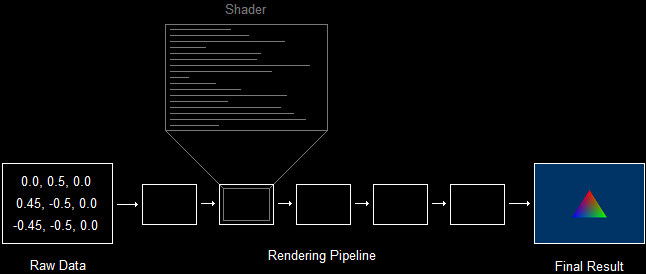There are several different types of shaders, and each is run many times during rendering. For example, a vertex shader is a program that is run once for each vertex rendered, while a pixel shader is a program that is run for each pixel drawn.

We will get to programming shaders in the next lesson. Right now, we are trying to render a triangle, and to do that we must load certain shaders to the GPU.

Each of these steps are very simple. Let's go over them quickly.

In this first part, we are going to compile two shaders. These are the vertex shader and the pixel shader, the two shaders that are required for rendering.

To load and compile a shader, we use a function called D3DX11CompileFromFile(). This function has a large number of parameters, a surprising number of which we can set to 0 and ignore until later.

Let's start by examining the prototype for this function:

HRESULT D3DX11CompileFromFile(
LPCTSTR pSrcFile,                // file containing the code
LPCSTR pFunctionName,            // name of the shader's starting function
LPCSTR pProfile,                 // profile of the shader

I'm going to walk through all the parameters, but ignore the ones labelled "advanced".

LPCSTSTR pSrcFile,

This first parameter is the name of the file containing the uncompiled shader code. For us, this is going to be L"shaders.shader".

Shaders are typically stored in files with the extention .shader, though you can use any extension you like.

LPCSTR pFunctionName,

LPCSTR pProfile,

A shader profile is a code that tells the compiler what type of shader we are compiling and what version to compile to. The code is formated like this:

vs_4_0

where "v" stands for vertex, "s" stands for shader, and "_4_0" stands for HLSL version 4.0. You can replace the "v" with a "p" for pixel shader.

The first time we call this function we'll use "vs_4_0" and the second time we'll use "ps_4_0".

This parameter is a pointer to a blob object. This blob object will be filled with the compiled code of the shader.

A blob object is a neat little COM object that stores a buffer of data. We can use the functions GetBufferPointer() and GetBufferSize() to access the contents. We'll see an example of this below.

Let's take a look at the function when written out in real code. It's actually quite simple:

ID3D10Blob *VS, *PS;

So what does this do exactly? For the vertex shader, it loads the contents of "shaders.shader", finds the function "VShader", compiles it as a version 4.0 vertex shader, and stores the compiled result in the blob VS.

This piece of code is going to grow, so let's place it into a new function called InitPipeline():

void InitPipeline()
{
ID3D10Blob *VS, *PS;
}

That said, let's find out what we can do with this compiled code.

Each shader is stored in its own COM object. As you may have gathered, we're interested in creating a vertex shader and a pixel shader. The COM objects for these are called ID3D11_____Shader:

// global

Once we have our pointers, we create the objects using dev->Create_____Shader(), like this:

void InitPipeline()
{
ID3D10Blob *VS, *PS;

}

There are four parameters here, three of which are obvious. The first is the address of the compiled data. The second is the size of the file data. The fourth is the address of the shader object.

The third is advanced and will be covered later.

This step is simple. It looks like this:

void InitPipeline()
{
ID3D10Blob *VS, *PS;

}

For these functions, the first parameter is the address of the shader object to set, while the othe two objects are advanced and will be covered later.

Remember that pVS and pPS are both COM objects, so they must be released.

void CleanD3D(void)
{
swapchain->SetFullscreenState(FALSE, NULL);    // switch to windowed mode

// close and release all existing COM objects
pVS->Release();
pPS->Release();
swapchain->Release();
backbuffer->Release();
dev->Release();
devcon->Release();
}

In summary, this function prepares our GPU for rendering. It has been given all the instructions it needs to turn vertices into a rendered image. Now all we need are some vertices.

Let's get to it!

Vertex Buffers

If you went through Lesson 1 in any great detail, you will recall the definition of vertex: the location and properties of an exact point in 3D space. The location simply consists of three numerical values which represent the vertex's coordinates. The properties of the vertex are also defined using numerical values.

Direct3D uses what is called an input layout. An input layout is the layout of the data containing the location and properties of a vertex. It would be a format of data that you can modify and set according to your needs. Let's take a look at how this works exactly.

A vertex is made of a struct, which contains the data pertinent to creating whatever 3D image it is made for. To display the image, we will copy all the information to the GPU and then order Direct3D to render the data to the back buffer. However, what if we were forced to send all the data that could possibly be wanted for a vertex? This would happen.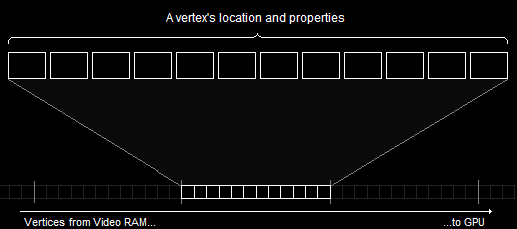A Vertex Format Containing All Possible Data

Of course, you may not see right away what the problem is here, but let's say we only needed two of these blocks of information. We could send it to the GPU much faster by doing it like this: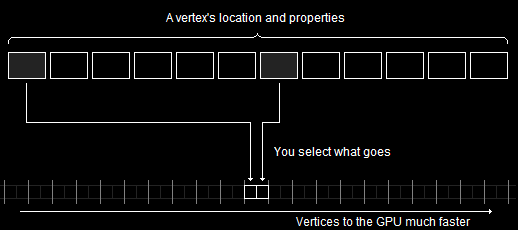A Selective Vertex Format Goes Faster

This is what happens when we use an input layout. We select which information we want to use, and send just that, enabling us to send many more vertices between each frame.

Creating Vertices

Let's start simple, and make a single vertex.

Vertices are typically created using a struct. In the struct you can place any data about the struct you like, in any format you like. For example, if we wanted each vertex to contain a position and a color, we could build the following struct:

struct VERTEX
{
FLOAT X, Y, Z;      // position
D3DXCOLOR Color;    // color
};

As you can see, we have three floats representing the position, and one representing the color.

Now let's build an actual vertex using this struct. We could do it like this:

VERTEX OurVertex = {0.0f, 0.5f, 0.0f, D3DXCOLOR(1.0f, 0.0f, 0.0f, 1.0f)};

Of course, we could also make an array of vertices like this:

VERTEX OurVertices[] =
{
{0.0f, 0.5f, 0.0f, D3DXCOLOR(1.0f, 0.0f, 0.0f, 1.0f)},
{0.45f, -0.5, 0.0f, D3DXCOLOR(0.0f, 1.0f, 0.0f, 1.0f)},
{-0.45f, -0.5f, 0.0f, D3DXCOLOR(0.0f, 0.0f, 1.0f, 1.0f)}
};

This results in a triangle, which we will seen drawn on the screen shortly.

Creating a Vertex Buffer

When creating a struct in C++ the data is stored in system memory, but we need it to be in video memory, which we don't have easy access to.

In order to allow us access to the video memory, Direct3D provides us with a specfic COM object that will let us maintain a buffer in both system and video memory.

How do buffers exist in both system and video memory? Well, initially the data in such a buffer will be stored in system memory. When rendering calls for it, Direct3D will automatically copy it over to video memory for you. If the video card becomes low on memory, Direct3D will delete buffers that haven't been used in a while, or are considered "low priority", in order to make room for newer resources.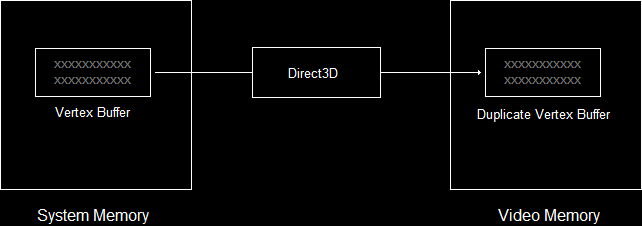Vertex Buffer in Video Memory When Needed

Why do we need Direct3D to do this for us? Well, it's very difficult to do this on your own, as accessing video memory varies depending on the video card and operating system version. Having Direct3D manage this for us is very, very handy.

This COM object is called ID3D11Buffer. To create it, we use the CreateBuffer() function. Here's what the code looks like:

ID3D11Buffer *pVBuffer;    // global

D3D11_BUFFER_DESC bd;
ZeroMemory(&bd, sizeof(bd));

bd.Usage = D3D11_USAGE_DYNAMIC;                // write access access by CPU and GPU
bd.ByteWidth = sizeof(VERTEX) * 3;             // size is the VERTEX struct * 3
bd.BindFlags = D3D11_BIND_VERTEX_BUFFER;       // use as a vertex buffer
bd.CPUAccessFlags = D3D11_CPU_ACCESS_WRITE;    // allow CPU to write in buffer

dev->CreateBuffer(&bd, NULL, &pVBuffer);       // create the buffer

Here's what it all means.

D3D11_BUFFER_DESC bd;

This is a struct containing properties of the buffer.

ZeroMemory(&bd, sizeof(bd));

As with many Direct3D structs, we need to initialize it by zeroing it out.

bd.Usage

Buffers are set up to be as efficient as possible. To do this correctly, Direct3D needs to know how we intend to access it.

This table shows the various flags that can be used here. We'll be using dynamic usage for this tutorial.

FlagCPU AccessGPU Access

bd.ByteWidth

This value contains the size of the buffer that will be created. This is the same size as the array of vertices we intend to put into it. For this lesson, we get this by calculating: sizeof(VERTEX) * 3.

bd.BindFlags

This value tells Direct3D what kind of buffer to make. There are several types of buffers we could make, but the kind we want to make is a vertex buffer. To do this, we can simply use the flag D3D11_BIND_VERTEX_BUFFER.

We won't go over the other flags here, as they are a bit advanced for this lesson. However, we will cover them all in due time.

bd.CPUAccessFlags

This member adds clarification to the usage flags, by telling Direct3D how we plan to access the CPU. The only flags here are D3D11_CPU_ACCESS_WRITE and D3D11_CPU_ACCESS_READ, and they can only be used in alignment with Table 2.1.

We will use D3D11_CPU_ACCESS_WRITE, because we want to copy data from system memory into the buffer.

dev->CreateBuffer(&bd, NULL, &pVBuffer);

The function that creates the buffer. The first parameter is the address of the description struct. The second parameter can be used to initialize the buffer with certain data upon creation, but we've set it to NULL here. The third parameter is the address of the buffer object. Here, pVBuffer means pointer to vertex buffer.

Filling the Vertex Buffer

So we now have some vertices for a triangle, and we have a vertex buffer to place them in. Now all we need to do is copy the vertices into the buffer.

However, because Direct3D may be working with a buffer in the background, it never gives you direct CPU access to it. In order to access it, the buffer must be mapped. This means that Direct3D allows anything being done to the buffer to finish, then blocks the GPU from working with the buffer until it is unmapped.

So to fill the vertex buffer we:

1. Map the vertex buffer (and thereby obtain the buffer's location).
2. Copy the data to the buffer (using memcpy()).
3. Unmap the buffer.

Here's how this looks in code:

D3D11_MAPPED_SUBRESOURCE ms;
devcon->Map(pVBuffer, NULL, D3D11_MAP_WRITE_DISCARD, NULL, &ms);   // map the buffer
memcpy(ms.pData, OurVertices, sizeof(OurVertices));                // copy the data
devcon->Unmap(pVBuffer, NULL);                                     // unmap the buffer

D3D11_MAPPED_SUBRESOURCE ms;

This is a struct which will be filled with information about the buffer once we've mapped it. This information will include a pointer to the buffer's location, and we can access this pointer using "ms.pData".

devcon->Map()

The next line maps the buffer, allowing us to access it. The parameters are pretty easy.

The first one is the address of the buffer object. Our buffer pointer is called pVBuffer, so use that.

The second one is advanced. We'll get to it later. Fortunately, we can set it to NULL for now.

The third parameter is a set of flags that allows us to control the CPUs access to the buffer while it's mapped. We are going to use D3D11_MAP_WRITE_DISCARD, but the others can be found in this table.

FlagDescription
DXD11_MAP_WRITEBuffer can only be written to by the CPU.
DXD11_MAP_WRITE_DISCARDPrevious contents of buffer are erased, and new buffer is opened for writing.
DXD11_MAP_WRITE_NO_OVERWRITEAn advanced flag that allows you to add more data to the buffer even while the GPU is using parts. However, you must not work with the parts the GPU is using.

The fourth parameter is another flag. It can be NULL or D3D11_MAP_FLAG_DO_NOT_WAIT. This flag forces the program to continue, even if the GPU is still working with the buffer.

The last parameter is the address of a D3D11_MAPPED_SUBRESOURCE struct. The function fills out the struct we designate here, giving us the info we need.

memcpy()

Next, we do a standard memcpy(). We use ms.pData as the destination, OurVertices (or whatever) as the source, and sizeof(OurVertices) as the size.

devcon->Unmap()

Last, we unmap the buffer. This reallows the GPU access to the buffer, and reblocks the CPU. The first parameter is the buffer (pVBuffer), and the second parameter is advanced (NULL).

There was a lot of new code covered in this section. To wrap it all up and make it simpler, I've placed all this code into a separate function called InitGraphics(). The entire function looks like this:

struct VERTEX{FLOAT X, Y, Z; D3DXCOLOR Color;};    // a struct to define a vertex
ID3D11Buffer *pVBuffer;                            // the vertex buffer

void InitGraphics()
{
// create a triangle using the VERTEX struct
VERTEX OurVertices[] =
{
{0.0f, 0.5f, 0.0f, D3DXCOLOR(1.0f, 0.0f, 0.0f, 1.0f)},
{0.45f, -0.5, 0.0f, D3DXCOLOR(0.0f, 1.0f, 0.0f, 1.0f)},
{-0.45f, -0.5f, 0.0f, D3DXCOLOR(0.0f, 0.0f, 1.0f, 1.0f)}
};

// create the vertex buffer
D3D11_BUFFER_DESC bd;
ZeroMemory(&bd, sizeof(bd));

bd.Usage = D3D11_USAGE_DYNAMIC;                // write access access by CPU and GPU
bd.ByteWidth = sizeof(VERTEX) * 3;             // size is the VERTEX struct * 3
bd.BindFlags = D3D11_BIND_VERTEX_BUFFER;       // use as a vertex buffer
bd.CPUAccessFlags = D3D11_CPU_ACCESS_WRITE;    // allow CPU to write in buffer

dev->CreateBuffer(&bd, NULL, &pVBuffer);       // create the buffer

// copy the vertices into the buffer
D3D11_MAPPED_SUBRESOURCE ms;
devcon->Map(pVBuffer, NULL, D3D11_MAP_WRITE_DISCARD, NULL, &ms);    // map the buffer
memcpy(ms.pData, OurVertices, sizeof(OurVertices));                 // copy the data
devcon->Unmap(pVBuffer, NULL);                                      // unmap the buffer
}

This is a key part of 3D programming, and we'll be using it and modifying quite a bit as we go on. At this point I would suggest going over this section a couple times to make sure you really get it.

Verifying the Input Layout

So far in this lesson we have

B) created a shape using vertices and readied them for the GPU to use.

You may be wondering how the GPU is capable of reading our vertices, when we placed them in our own, self-created struct. How can it know that we placed location before color? How can it know we didn't mean something else?

The answer is the input layout.

As mentioned before, we are able to select what information gets stored with each vertex in order to improve the speed of rendering. The input layout is an object which contains the vertex struct's layout, letting the GPU organize the data appropriately and efficiently.

The ID3D11InputLayout object stores the layout of our VERTEX struct. To create this object we call the CreateInputLayout() function.

There are two parts to this. First, we need to define each element of the vertex. Second, we need to create the input layout object. line

Create the Input Elements

A vertex layout consists of one or more input elements. An input element is one property of the vertex, such as position or color.

Each element is defined by a struct, called D3D11_INPUT_ELEMENT_DESC. This struct describes a single vertex property.

To create a vertex with multiple properties, we just put these structs into an array.

D3D11_INPUT_ELEMENT_DESC ied[] =
{
{"POSITION", 0, DXGI_FORMAT_R32G32B32_FLOAT, 0, 0, D3D11_INPUT_PER_VERTEX_DATA, 0},
{"COLOR", 0, DXGI_FORMAT_R32G32B32A32_FLOAT, 0, 12, D3D11_INPUT_PER_VERTEX_DATA, 0},
};

There are seven values in this struct.

The first value is called the semantic. A semantic is a string which tells the GPU what the value is used for. This table shows a few semantic values, and we'll take up more as we go on.

SemanticValues
POSITIONfloat, float, float - or - D3DXVECTOR3
POSITIONTfloat, float, float - or - D3DXVECTOR3
COLORfloat, float, float, float - or - D3DXVECTOR4 - or - D3DXCOLOR
PSIZEfloat

The second value is the semantic index. If we had a vertex that had two colors, they would both use the COLOR semantic. To avoid confusion, we would have each property have a different number here.

The third value is the format of the data. On many semantics, the number of values is arbitrary (so long as its less than four). All that matters is that the format matches what you use in your vertices.

The fourth value is called the input slot. This is advanced, and we'll cover it later. For now, it should be 0.

The fifth value indicates how many bytes into the struct the element is. This is called the offset. As you can see, position has an offset of 0 and the color has an offset of 12. This means the position starts 0 bytes into the struct and the color starts 12 bytes into the struct. Actually, you can just put D3D11_APPEND_ALIGNED_ELEMENT and it will figure it out for you, but I ran out of room. :(

The sixth value is what the element is used as. There are two possible flags here, but the one we are interested in is D3D11_INPUT_PER_VERTEX_DATA. We'll cover the other flag later.

The last value is not used with the D3D11_INPUT_PER_VERTEX_DATA flag, so we'll have it be 0.

Create the Input Layout Object

This is the last thing before we get to start drawing. I promise.

Here, we call CreateInputLayout(), and thus create an object representing the vertex format. Let's look at the prototype for the function before we discuss it.

HRESULT CreateInputLayout(
D3D11_INPUT_ELEMENT_DESC *pInputElementDescs,
UINT NumElements,
SIZE_T BytecodeLength,
ID3D11InputLayout **pInputLayout);

This is actually one of the easier functions in Direct3D, despite its appearance.

D3D11_INPUT_ELEMENT_DESC *pInputElementDescs,

This first parameter is a pointer to the element description array. We'll put &ied here.

UINT NumElements,

Pretty self-explanatory, this parameter is the number of elements in the array. For us this is 2.

Whoa! This is the pointer to the first shader in the pipeline, which is the vertex shader. This means we put 'VS->GetBufferPointer()' here.

SIZE_T BytecodeLength,

This is the length of the shader file. We can just put 'VS->GetBufferSize()' here.

ID3D11InputLayout **pInputLayout

This is the pointer to the input layout object. We might as well call it something simple, like 'pLayout', so we'll put '&pLayout' here.

Here's how the function actually looks when written out:

ID3D11InputLayout *pLayout;    // global

D3D11_INPUT_ELEMENT_DESC ied[] =
{
{"POSITION", 0, DXGI_FORMAT_R32G32B32_FLOAT, 0, 0, D3D11_INPUT_PER_VERTEX_DATA, 0},
{"COLOR", 0, DXGI_FORMAT_R32G32B32A32_FLOAT, 0, 12, D3D11_INPUT_PER_VERTEX_DATA, 0},
};

dev->CreateInputLayout(ied, 2, VS->GetBufferPointer(), VS->GetBufferSize(), &pLayout);

Of course, to run this function, we'll need access to VSFile and VSSize, so we'll place this code right in the InitPipeline() function.

void InitPipeline()
{
ID3D10Blob *VS, *PS;

// create the input layout object
D3D11_INPUT_ELEMENT_DESC ied[] =
{
{"POSITION", 0, DXGI_FORMAT_R32G32B32_FLOAT, 0, 0, D3D11_INPUT_PER_VERTEX_DATA, 0},
{"COLOR", 0, DXGI_FORMAT_R32G32B32A32_FLOAT, 0, 12, D3D11_INPUT_PER_VERTEX_DATA, 0},
};

dev->CreateInputLayout(ied, 2, VS->GetBufferPointer(), VS->GetBufferSize(), &pLayout);

}

One last thing before we move on. Creating the input layout does nothing until it has been set. To set the input layout we call the IASetInputLayout() function. Its only parameter is the input layout object.

void InitPipeline()
{
ID3D10Blob *VS, *PS;

// create the input layout object
D3D11_INPUT_ELEMENT_DESC ied[] =
{
{"POSITION", 0, DXGI_FORMAT_R32G32B32_FLOAT, 0, 0, D3D11_INPUT_PER_VERTEX_DATA, 0},
{"COLOR", 0, DXGI_FORMAT_R32G32B32A32_FLOAT, 0, 12, D3D11_INPUT_PER_VERTEX_DATA, 0},
};

dev->CreateInputLayout(ied, 2, VS->GetBufferPointer(), VS->GetBufferSize(), &pLayout);
devcon->IASetInputLayout(pLayout);
}

Now let's actually draw something!

Drawing the Primitive

There are three simple functions that we have call to do rendering.

The first one sets which vertex buffer we intend to use. The second sets which type of primitive we intend to use (such as triangle lists, line strips, etc.) The third actually draws the shape.

IASetVertexBuffers()

The first of these functions is IASetVertexBuffers(). This will tell the GPU which vertices to read from when rendering. It has a couple of easy parameters, so let's look at the protoype:

void IASetVertexBuffers(UINT StartSlot,
UINT NumBuffers,
ID3D11Buffer **ppVertexBuffers,
UINT *pStrides,
UINT *pOffsets);

The first parameter is advanced, so we'll set it to 0 for now.

The second parameter tells how many buffers we are setting. Because we only have one buffer, we'll put 1 here.

The third parameter is a pointer to an array of vertex buffers. We only have one, so we can fill this with &pVBuffer.

The fourth parameter points to an array of UINTs, which tell the sizes of a single vertex in each vertex buffer. To fill this parameter we create a UINT, fill it with "sizeof(VERTEX)", and put the address of that UINT here.

The fifth parameter is an array of UINTs telling the number of bytes into the vertex buffer we should start rendering from. This will usually be 0. To do this, we create a UINT of 0 and put the address here.

It's a fairly simple function. Here's what it looks like:

UINT stride = sizeof(VERTEX);
UINT offset = 0;
devcon->IASetVertexBuffers(0, 1, &pBuffer, &stride, &offset);

IASetPrimitiveTopology()

This second function tells Direct3D which type of primitive that is used. These were covered in Lesson 3, but the codes used are here:

FlagDescription
D3D11_PRIMITIVE_TOPOLOGY_POINTLISTShows a series of points, one for each vertex.
D3D11_PRIMITIVE_TOPOLOGY_LINELISTShows a series of separated lines.
D3D11_PRIMITIVE_TOPOLOGY_LINESTRIPShows a series of connected lines.
D3D11_PRIMITIVE_TOPOLOGY_TRIANGLELISTShows a series of separated triangles.
D3D11_PRIMITIVE_TOPOLOGY_TRIANGLESTRIPShows a series of connected triangles.

The only parameter of this function is one of these flags. It is written out like this:

devcon->IASetPrimitiveTopology(D3D11_PRIMITIVE_TOPOLOGY_TRIANGLELIST);

Draw()

Now that we have told Direct3D what kind of primitives to render, and what vertex buffer to read from, we tell it to draw the contents of the vertex buffer.

This function draws the primitives in the vertex buffer to the back buffer. Here is the prototype:

void Draw(UINT VertexCount,             // the number of vertices to be drawn
UINT StartVertexLocation);    // the first vertex to be drawn

These parameters control which vertices in the buffer to draw. The second parameter is a number telling the first vertex in the buffer that should be drawn, while the first parameter is the number of vertices that should be drawn.

In practice, the function looks like this:

devcon->Draw(3, 0);    // draw 3 vertices, starting from vertex 0

Now let's take a look at the entire render_frame() function now that we have modified it.

// this is the function used to render a single frame
void RenderFrame(void)
{
// clear the back buffer to a deep blue
devcon->ClearRenderTargetView(backbuffer, D3DXCOLOR(0.0f, 0.2f, 0.4f, 1.0f));

// select which vertex buffer to display
UINT stride = sizeof(VERTEX);
UINT offset = 0;
devcon->IASetVertexBuffers(0, 1, &pVBuffer, &stride, &offset);

// select which primtive type we are using
devcon->IASetPrimitiveTopology(D3D10_PRIMITIVE_TOPOLOGY_TRIANGLELIST);

// draw the vertex buffer to the back buffer
devcon->Draw(3, 0);

// switch the back buffer and the front buffer
swapchain->Present(0, 0);
}

A Quick Review

Because a lot of code was covered in this lesson, let's stop and take a quick review of the whole thing. We'll start at the beginning and walk through everything again.

Shaders are mini-programs which are used to direct the GPU in rendering. Rendering is impossible without shaders. In the first section, we loaded two shaders and loaded them up to the GPU.

The function, InitPipeline() looked like this:

void InitPipeline()
{
ID3D10Blob *VS, *PS;

}

In here we:

2. Created objects for each one.
3. Set the two objects.

Vertex Buffers

In this section we learned to create a struct representing a vertex, and to create a Vertex Buffer object that handled the vertices in video memory. We built a function called InitGraphics().

// global
struct VERTEX{FLOAT X, Y, Z; D3DXCOLOR Color;};    // a struct to define a vertex
ID3D11Buffer *pVBuffer;                            // the vertex buffer

void InitGraphics()
{
// create a triangle using the VERTEX struct
VERTEX OurVertices[] =
{
{0.0f, 0.5f, 0.0f, D3DXCOLOR(1.0f, 0.0f, 0.0f, 1.0f)},
{0.45f, -0.5, 0.0f, D3DXCOLOR(0.0f, 1.0f, 0.0f, 1.0f)},
{-0.45f, -0.5f, 0.0f, D3DXCOLOR(0.0f, 0.0f, 1.0f, 1.0f)}
};

// create the vertex buffer
D3D11_BUFFER_DESC bd;
ZeroMemory(&bd, sizeof(bd));

bd.Usage = D3D11_USAGE_DYNAMIC;                // write access access by CPU and GPU
bd.ByteWidth = sizeof(VERTEX) * 3;             // size is the VERTEX struct * 3
bd.BindFlags = D3D11_BIND_VERTEX_BUFFER;       // use as a vertex buffer
bd.CPUAccessFlags = D3D11_CPU_ACCESS_WRITE;    // allow CPU to write in buffer

dev->CreateBuffer(&bd, NULL, &pVBuffer);       // create the buffer

// copy the vertices into the buffer
D3D11_MAPPED_SUBRESOURCE ms;
devcon->Map(pVBuffer, NULL, D3D11_MAP_WRITE_DISCARD, NULL, &ms);    // map the buffer
memcpy(ms.pData, OurVertices, sizeof(OurVertices));                 // copy the data
devcon->Unmap(pVBuffer, NULL);                                      // unmap the buffer
}

In this function we:

1. Created three vertices with both position and color.
2. Created a vertex buffer object.
3. Copied the vertices into the vertex buffer by mapping it, copying the data, and unmapping it.

Verifying the Input Layout

In this section we covered how to coordinate the vertex buffer and the shader by using an input layout object. There was no separate function for this, but we placed the code in the InitPipeline() function, shown in bold below:

void InitPipeline()
{
ID3D10Blob *VS, *PS;

// create the input layout object
D3D11_INPUT_ELEMENT_DESC ied[] =
{
{"POSITION", 0, DXGI_FORMAT_R32G32B32_FLOAT, 0, 0, D3D11_INPUT_PER_VERTEX_DATA, 0},
{"COLOR", 0, DXGI_FORMAT_R32G32B32A32_FLOAT, 0, 12, D3D11_INPUT_PER_VERTEX_DATA, 0},
};

dev->CreateInputLayout(ied, 2, VS->GetBufferPointer(), VS->GetBufferSize(), &pLayout);
devcon->IASetInputLayout(pLayout);

}

Here's all we did:

1. Created input element descriptions for the position and the color.
2. Created an input layout object using the shader information.
3. Set the input layout object.

Drawing the Primitive

Finally we actually got to draw the triangle. We added a few lines of code to the RenderFrame() function.

// this is the function used to render a single frame
void RenderFrame(void)
{
// clear the back buffer to a deep blue
devcon->ClearRenderTargetView(backbuffer, D3DXCOLOR(0.0f, 0.2f, 0.4f, 1.0f));

// select which vertex buffer to display
UINT stride = sizeof(VERTEX);
UINT offset = 0;
devcon->IASetVertexBuffers(0, 1, &pVBuffer, &stride, &offset);

// select which primtive type we are using
devcon->IASetPrimitiveTopology(D3D10_PRIMITIVE_TOPOLOGY_TRIANGLELIST);

// draw the vertex buffer to the back buffer
devcon->Draw(3, 0);

// switch the back buffer and the front buffer
swapchain->Present(0, 0);
}

In here we:

1. Set which vertex buffer to use (there was only one to choose from).
2. Set which primitive type to use.
3. Draw the triangle.

The Finished Program

Before you build and run this program, you will need the shader file itself. It is called "Shaders.shader", and the contents can be found where it says "Show Shaders" below. At this point, you won't necessarily understand the shader code. We'll be covering it in detail in the next tutorial.

This file must be saved into the project folder itself. If you select 'Show All Files' in your Solution Explorer, you should be able to see it listed. If you don't, it is in the wrong place and you will not be able to run the program. It should look like this: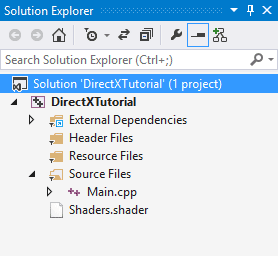The Shader File in Solution Explorer

Let's examine the final DirectX code. The new parts covered in this lesson are in bold as usual.

Go ahead and update your program and let's see what we get. If you run this, you should see the following on your screen: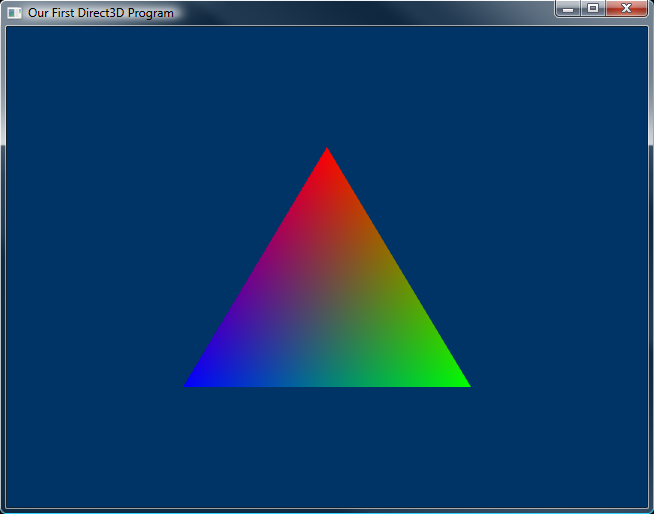The Rendered Triangle

Well, this lesson covered quite a big step. There are many little parts to it, not all of which may seem relevant. In order to get a better idea of what's happening, answer these questions and do these exercises. They'll get you ready for what's to come.

Questions

1. What is a shader and what does it do?
2. What is a vertex buffer?
3. How do input layouts make vertex buffers more efficient?

Exercises

1. Change the colors and shape of the triangle.
2. In earlier lessons, changing the viewport did nothing. See what it does now.
3. Render using lines or points.

Next Lesson: The Rendering Pipeline

GO! GO! GO!